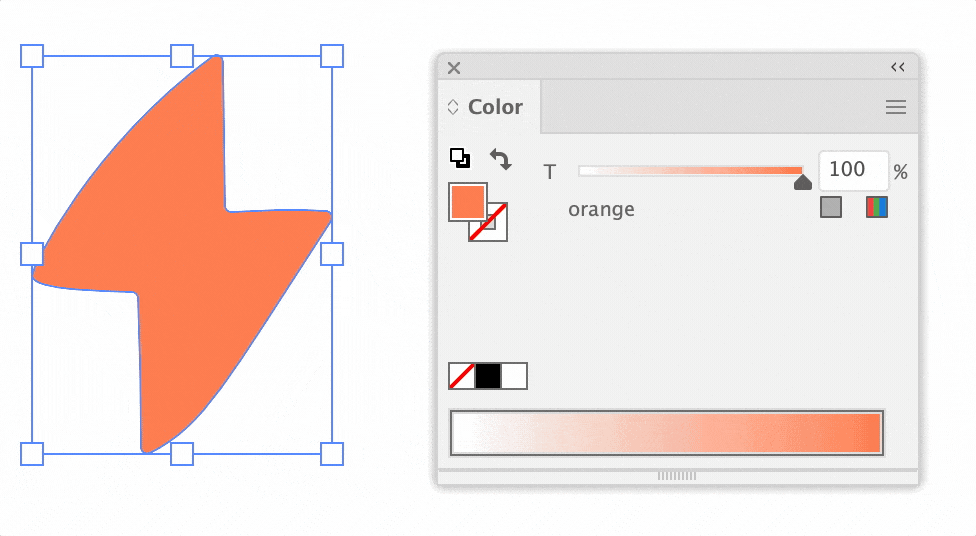# Get SpotColor tint colorThe color values are the same for different the spot tint

## Parsing method

The global color tint in Illustrator is created by linear interpolation. It is like a gradient from white to a given color. Therefore, an interpolation function is sufficient to find the value.

`function lerp(start, end, t) {  return start + (end - start) * t;}`
• end — 100% spot color value
• t — percentage in the range 0–1

## Sample script

`var isRgbDoc = activeDocument.documentColorSpace === DocumentColorSpace.RGB,    start, end = selection.fillColor,    tintVal = []; // Array for color informationif (end.typename === 'SpotColor') { // Script parse spot colors  // Initialize the starting white color  if (isRgbDoc) {    start = new RGBColor();    start.red = 255;    start.green = 255;    start.blue = 255;  } else {    start = new CMYKColor();    start.cyan = 0;    start.magenta = 0;    start.yellow = 0;    start.black = 0;  }  var t = end.tint / 100;  end = end.spot.color;  // Apply lerp() to each channel in the color object  for (var key in end) {    if (typeof end[key] === 'number') {      tintVal.push( lerp(start[key], end[key], t) );    }  }  alert(isRgbDoc ? 'RGB : ' + tintVal : 'CMYK : ' + tintVal);}function lerp(start, end, t) {  return start + (end - start) * t;}`